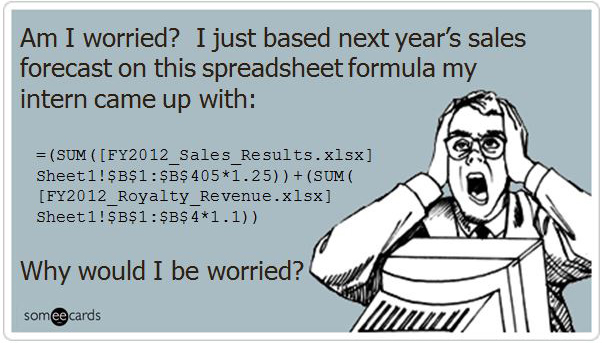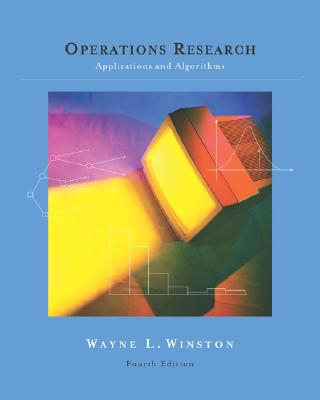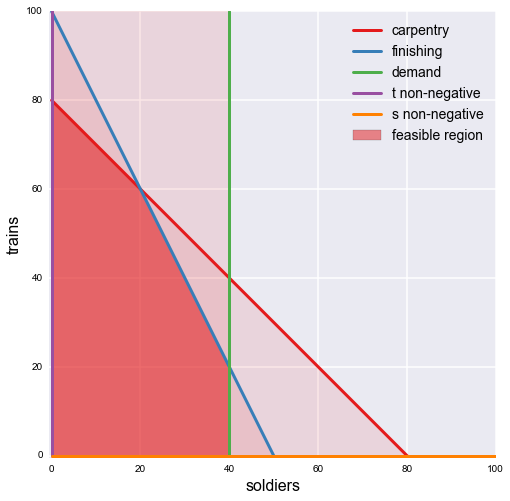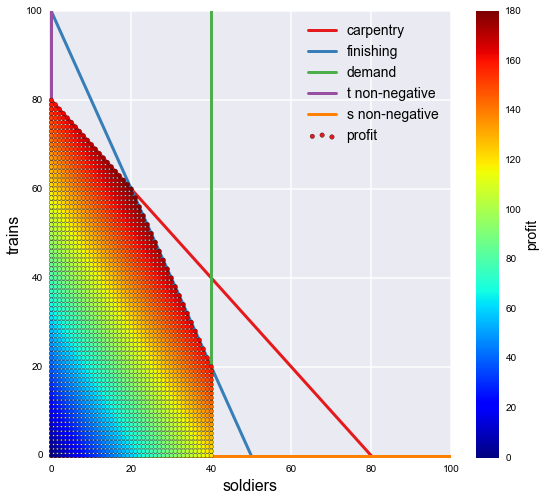## What is the right tool for LP modeling?¶

Most software developers I know hate Excel and argue that it should be avoided. This position is understandable if you have ever seen the typical bug-ridden, unofficial, magic-number-filled, garishly colored, copy-pasted, formula-hell spreadsheets that tend to float around organizations, passed like mystical talismans from one generation to the next. (Let's not even talk about the horrors involved with amateur VBA-extended spreadsheets.) Something about spreadsheets seems to make them especially prone to certain kinds of errors, even when used by people who know what they're doing.Even so, there is definitely something to be said for a well crafted, nicely formatted spreadsheet model for an optimization problem. First of all, it's visual; you can see all of the problem components at the same time. Second, it is interactive; you can play with your decision variables and get immediate feedback when all of the dependent cells automatically recalculate. Third — and this is something that academics and those of us used to using open source tools often forget — Excel and its Solver extension are ubiquitous and pre-approved for use, even in the average user's workstation in a large organization with byzantine enterprise IT policies.

The real problem is that spreadsheet models do not scale well past a certain level of complexity, beyond which they are impossible to fully understand (and therefore impossible to trust). Imagine a routing problem where we have thousands of nodes and some metadata about them such as demand or supply or capacity, and that we need to enter a number of constraints for each node based on this metadata and some complicated business rules. Using a spreadsheet would either mean doing this by hand (no thanks), or incorporating some kind of large lookup table into the already verbose and hard to read spreadsheet formulas. That sounds terrible. So what should we use instead?What manner of black magic is this?

LP/ILP modeling is not hard using a modeling language like AMPL, but there can be a lot of cognitive overhead in switching to an entirely different language, especially if what we're doing is not just one ad hoc analysis but is part of a larger, continuing workflow. For those of us that already spend a lot of time in Python, it would be nice to do our optimization work in the same language we are already using on either end of the problem. Luckily, we can use one of the many packages designed for precisely this purpose, such as pulp, PyGLPK, or PyMathProg.

"But Python is sloooooow!!!1"

Good thing we're only using it to set up the problem! The hard work is actually done by the solver package of your choice.

## An example problem using Python¶

Let's use one of these packages to demonstrate an example from Winston. I'll choose pulp since it has good documentation and an excellent starter paper, and I've used it before in another project:Example 3.1

Giapetto’s Woodcarving, Inc., manufactures two types of wooden toys: soldiers and trains.

A soldier sells for 27 dollars and uses 10 dollars worth of raw materials. Each soldier that is manufactured increases Giapetto’s variable labor and overhead costs by 14 dollars. A train sells for 21 dollars and uses 9 dollars worth of raw materials. Each train built increases Giapetto’s variable labor and overhead costs by 10 dollars. The manufacture of wooden soldiers and trains requires two types of skilled labor: carpentry and finishing. A soldier requires 2 hours of finishing labor and 1 hour of carpentry labor. A train requires 1 hour of finishing labor and 1 hour of carpentry labor. Each week, Giapetto can obtain all the needed raw material but only 100 finishing hours and 80 carpentry hours. Demand for trains is unlimited, but at most 40 soldiers are bought each week. Giapetto wants to maximize weekly profit (revenues-costs).

Formulate a mathematical model of Giapetto’s situation that can be used to maximize Giapetto’s weekly profit.

First, we'll import the necessary packages and create the pulp LP object we'll be working with.

In :
import numpy as np
import pulp

# create the LP object, set up as a maximization problem
prob = pulp.LpProblem('Giapetto', pulp.LpMaximize)

# set up decision variables
soldiers = pulp.LpVariable('soldiers', lowBound=0, cat='Integer')
trains = pulp.LpVariable('trains', lowBound=0, cat='Integer')


Now we add in the objective function:

In :
# model weekly production costs
raw_material_costs = 10 * soldiers + 9 * trains
variable_costs = 14 * soldiers + 10 * trains

# model weekly revenues from toy sales
revenues = 27 * soldiers + 21 * trains

# use weekly profit as the objective function to maximize
profit = revenues - (raw_material_costs + variable_costs)
prob += profit  # here's where we actually add it to the obj function


And now the constraints:

In :
# add constraints for available labor hours
carpentry_hours = soldiers + trains
prob += (carpentry_hours <= 80)

finishing_hours = 2*soldiers + trains
prob += (finishing_hours <= 100)

# add constraint representing demand for soldiers
prob += (soldiers <= 40)


Let's print out the problem and make sure we have everything:

In :
print(prob)

Giapetto:
MAXIMIZE
3*soldiers + 2*trains + 0
SUBJECT TO
_C1: soldiers + trains <= 80

_C2: 2 soldiers + trains <= 100

_C3: soldiers <= 40

VARIABLES
0 <= soldiers Integer
0 <= trains Integer



Looks good — now we solve the LP.

In :
# solve the LP using the default solver
optimization_result = prob.solve()

# make sure we got an optimal solution
assert optimization_result == pulp.LpStatusOptimal

# display the results
for var in (soldiers, trains):
print('Optimal weekly number of {} to produce: {:1.0f}'.format(var.name, var.value()))

Optimal weekly number of soldiers to produce: 20
Optimal weekly number of trains to produce: 60


We can also visualize this problem and verify that the answer we have come up with makes sense. Here are the constraints:

$$s + t \leq 80$$$$2s + t \leq 100$$$$0 \leq s \leq 80$$$$t \geq 0$$$$s, t \in \mathbb{Z}$$

We can rewrite the ones with both $s$ and $t$ as follows so that they can be plotted as lines on a 2D graph with an $s$-axis and a $t$-axis:

$$t \leq 80 - s$$$$t \leq 100 - 2s$$

Let's graph all these simultaneous inequalities:

In :
%matplotlib inline
from matplotlib import pyplot as plt
from matplotlib.path import Path
from matplotlib.patches import PathPatch

# use seaborn to change the default graphics to something nicer
# and set a nice color palette
import seaborn as sns
sns.set_color_palette('Set1')

# create the plot object
fig, ax = plt.subplots(figsize=(8, 8))
s = np.linspace(0, 100)

# add carpentry constraint: trains <= 80 - soldiers
plt.plot(s, 80 - s, lw=3, label='carpentry')
plt.fill_between(s, 0, 80 - s, alpha=0.1)

# add finishing constraint: trains <= 100 - 2*soldiers
plt.plot(s, 100 - 2 * s, lw=3, label='finishing')
plt.fill_between(s, 0, 100 - 2 * s, alpha=0.1)

# add demains constraint: soldiers <= 40
plt.plot(40 * np.ones_like(s), s, lw=3, label='demand')
plt.fill_betweenx(s, 0, 40, alpha=0.1)

plt.plot(np.zeros_like(s), s, lw=3, label='t non-negative')
plt.plot(s, np.zeros_like(s), lw=3, label='s non-negative')

# highlight the feasible region
path = Path([
(0., 0.),
(0., 80.),
(20., 60.),
(40., 20.),
(40., 0.),
(0., 0.),
])
patch = PathPatch(path, label='feasible region', alpha=0.5)

# labels and stuff
plt.xlabel('soldiers', fontsize=16)
plt.ylabel('trains', fontsize=16)
plt.xlim(-0.5, 100)
plt.ylim(-0.5, 100)
plt.legend(fontsize=14)
plt.show()The highlighted area shows the set of decisions about $s$ and $t$ which satisfy all of the constraints — this is the feasible region. By inspection, this is clearly a convex polytope.

One possible complaint about this graphic is that it is a bit misleading. The solid feasible region seems to suggest a continuous area of possible choices for $s$ and $t$, but this is not the case. One last constraint is that the decision variables must be integers. Let's see if we can improve on the plot:

In :
fig, ax = plt.subplots(figsize=(9, 8))
s = np.linspace(0, 100)

# plot the constraints again
plt.plot(s, 80 - s, lw=3, label='carpentry')
plt.plot(s, 100 - 2 * s, lw=3, label='finishing')
plt.plot(40 * np.ones_like(s), s, lw=3, label='demand')
plt.plot(np.zeros_like(s), s, lw=3, label='t non-negative')
plt.plot(s, np.zeros_like(s), lw=3, label='s non-negative')

# plot the possible (s, t) pairs
pairs = [(s, t) for s in np.arange(101)
for t in np.arange(101)
if (s + t) <= 80
and (2 * s + t) <= 100
and s <= 40]

# split these into our variables
ss, ts = np.hsplit(np.array(pairs), 2)

# caculate the objective function at each pair
z = 3*ss + 2*ts  # the objective function

# plot the results
plt.scatter(ss, ts, c=z, cmap='jet', label='profit', zorder=3)

# labels and stuff
cb = plt.colorbar()
cb.set_label('profit', fontsize=14)
plt.xlabel('soldiers', fontsize=16)
plt.ylabel('trains', fontsize=16)
plt.xlim(-0.5, 100)
plt.ylim(-0.5, 100)
plt.legend(fontsize=14)
plt.show()

plt.show()This plot also shows that the optimal point is at a vertex as expected, while the clearly parallel stripes of color demonstrate linear decision frontier for different values of the objective function.

Having done this work, we can confidently tell Giapetto how many of each toy to sell in order to maximize his profits, and even show him how much he would stand to make at any of the other possible decision points.

### References:¶

 Winston, Wayne. Operations Research: Applications and Algorithms, 4rd ed. Belmont, CA: Duxbury Press, 2004.

 Mitchell, Stuart. "An introduction to pulp for Python programmers." Python Papers Monograph 1 (2009): 14.

Any comments or suggestions? Let me know.